# Pre-Algebra - Grade 7 and 8

Sale ends in
Teacher: Sheila
Customers Who Have Viewed This Course: 9513
\$200.00
\$95.00

#### 1 Course Introduction 05:27

I would like to introduce myself to you and share my background in education. I have been teaching for more than thirty-four years, and the majoriy of that time has been spent teaching mathematics. I have a Bachelor of Science degree in Elementary Education, a Master's Degree in Adult Education and Training, and an Education Specialist Degree in Education Leadership. I would love to help your child learn the basics of algebra and hopefully help your child feel confident in their skills and develop a love for mathematics and learning. Please try out the free videos and I hope that you find the course to be beneficial to helping your child be successful.

#### 2 Cornell Notes 04:14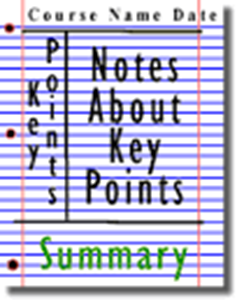Cornell University developed the Cornell Note Taking System to help students learn to take notes effectively and to use their notes effectively. The template has a very specific way for students to organize their notes that I explain in the video. After taking the notes, the system shows students how to effectively use their notes to help students retain the information and to develop a better understanding of the content. I use the Cornell Note System in all of my math classes and find it to be an extremely effective tool in helping students to learn the content, to find out what they do not understand, and to be more effective in asking questions about areas they do not fully understand.

#### 3 What Are Real Numbers, Really? 13:01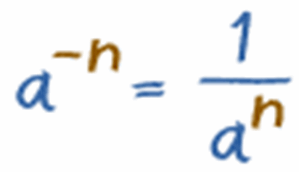Students will explore the real number system and its appropriate usage in real-world situations. Students will estimate and compare the value of irrational numbers by plotting them on a number line. Students will extend prior knowledge to translate among multiple representations of rational numbers (fractions, decimal numbers, percentages).

#### 4 Real Numbers Practice 04:24

Students can assess their mastery of Real Numbers by watching the video of the quiz and the correct answers.

#### 5 Rational Numbers 12:19Students will compare and estimate the value of rational and irrational numbers in different forms. Students will be able to plot rational numbers and irrational numbers on a number line. Students will understand the properties of numbers that make up the real number system and how they are used in the real world.

#### 6 Rational Numbers Practice 03:20

This is a review video of the assigned practice for Lesson 4, "Rational Numbers". Please use this video to evaluate your success with this lesson. Make sure that you correct any errors that you made and correct your mistakes making sure that you understand your mistake. I hope you were successful with the practice. If not, please take time to review any misunderstanding that you had.

#### 7 Fractions Decimals Percents 12:19Students will extend prior knowledge to translate among multiple representations of rational numbers (fractions, decimal numbers, percentages). Students will learn how to translate from any one of the three forms to another form and recognize the more common translations.

#### 8 Fractions to Percents Practice 08:30

In this lesson students converted from fractions to percents. Use the video to evaluate your practice. Pay close attention to any errors that you have and make certain that you understand why you made the error. This is the best strategy for mastering the content. Good luck!

#### 9 Fractions to Percents Practice Part Two 03:27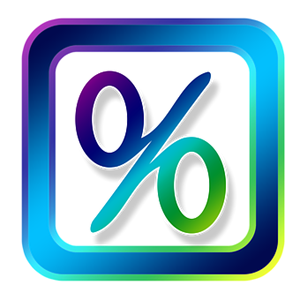Extend prior knowledge to translate among multiple representations of rational numbers (fractions, decimal numbers, percentages). Include the conversion of repeating decimal numbers to fractions. This lesson will focus on converting from fractions to percents and percents to fractions.

#### 10 Fractions Decimals and Percents Practice 05:46

This video reviews the practice provided for Lesson 4, "Fractions, Decimals, and Percents." Please use the video to check your practice and review any mistakes that you may have made. Remember, analyzing your own mistakes will increase your understanding of the properties for fractions, decimals, and percents. Here is hoping that you have done well.

#### 11 Fractions to Decimals Practice 02:41

In this practice, students are changing from fractions to decimals. Use the following video to review your practice work. Remember to always look at any errors you had and evaluate your mistakes. This is the best learning practice. Good luck, hoping you did GREAT!

#### 12 Finding Fractions by Long Division 07:23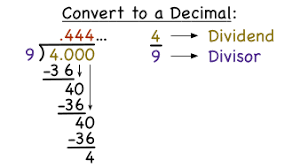Students will learn how to use long division to first find the equivalent decimal and then write the decimal as a fraction in simplest form.

#### 13 Fractions by Long Division Practice 07:29

This video shows students how to complete the practice for changing fractions to decimals using long division.

#### 14 Fractions by Long Division Practice 07:29

Students will use the video to assess their mastery of using long division to change fractions to decimals.

#### 15 Monomials 13:24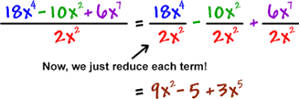Students will learn how to work with monomials within other numbers. Students leanr how to solve problems with like terms and different exponents. Mastering this skill also helps students to master the distributive property that becomes very important as students move to solving equations involving polynomials.

#### 16 Monomial Practice for adding and subtracting Practice I 07:29

Students will use the video to assess their mastery of adding and subtracting monomials.

#### 17 Monomial Practice For Multiplying and Dividing 04:56

Students will use the video to pactice their skills with solving monomials. Make sure that you evaluate any mistakes that you may have made and understand what your error was. Good Luck.

#### 18 Working with Exponents 09:17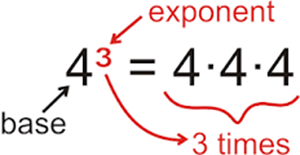Students will need to solve equations containing variables with different exponential notation. This lesson teaches students the laws of exponents and how to solve problems containing a variety of different exponential notations within one problem. Students must understand and aply the laws of exponents (i.e., product rule, quotient rule, power to a power, product to a power, quotient to a power, zero power property, negative exponents) to simplify numerical expressions that include integer exponents.

#### 19 Exponents Practice 03:45

Students will use the interactive quiz to assess the students' mastery of exponents. Good luck!

#### 20 Understanding Ratios 11:27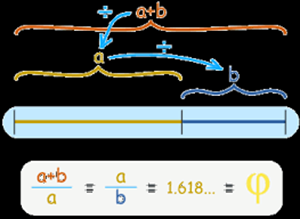Students will learn to read, write, and understand ratios. Students will be able to identify a ratio, rate, unit rate, and whether or not two ratios are proportional from a table or graph.

#### 21 Ratios Practice 06:42

Students will use the video to evaluate their solutions to ratio words problems. Remember to analyze your mistakes before moving on. Good Luck.

#### 22 Scientific Notation 12:57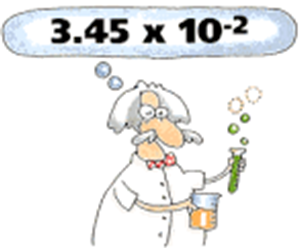Understand and apply the laws of exponents (i.e., product rule, quotient rule, power to a power, product to a power, quotient to a power, zero power property, negative exponents) to simplify numerical expressions that include integer exponents.

#### 23 Scientific Notation Practice Video 07:07

Students will use the video to evaluate their success with the Practice for Scientific Notation. Again, be sure to examine your errors and make certain that you understand your mistakes. Good Luck!

#### 24 Roots 15:42Students will investigate the concepts of square and cube roots. Students will learn how to solve problems with roots and understand their function in the distributive property.

#### 25 Roots Practice 04:59

Use the video to evaluate your practice on square and cube roots. Make sure to understand any errors that you made. Good luck.

#### 26 Proportional vs. Nonproportional 13:11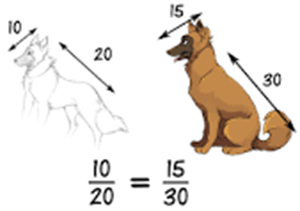Students will learn to identify proportional and non-proportional relationships through a variety of methods. This is a skill that will be used in a variety of mathematical topics studied in pre-algebra and algebra. Students will identify proportions using both tables and graphs.

#### 27 Proportional or Non-Proportional Practice 05:44

Students will use this video to evaluate their practice for proportional and non-proportional relationships. Be sure to evaluate any mistakes that you made and make sure you understand why your response was incorrect. Good luck.

#### 28 Properties of Numbers 13:43Students must develop a full understanding of the properties of real numbers. The properties are the building blocks of number sense and ultimately determine a student's ability to understand and solve problems. These are extremely important as a student begins to solve algebraic equations and study higher mathematics.

#### 29 Properties of Numbers Practice 02:57

Students need to understand the properties of numbers. This understanding of the properties enables students to make decisions on how to move numbers when solving equations. Without understanding the properties of numbers students struggle in solving multiple step equations.

#### 30 Practice Part II 02:50

Students will use the video to assess their practice for the lesson on Properties of Numbers.

#### 31 Pre-Algebra I 11:19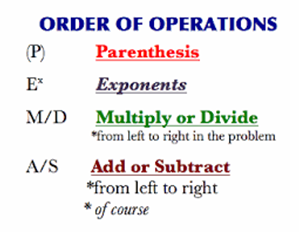Students will review the order of operations in preparation for solving equations. It is imperative that students master the order of operations before beginning to solve equations.

#### 31 Pre-Algebra I Part One Combining Like Terms Practice Part One 04:40

This video is of the practice for "Combining Llike Terms" which is divided into two videos. Please make certain that you use this video first to check your practice. If you have any errors, please take the time to evaluate your mistakes so that you have mastery of the content. Here is hoping you have done well!

#### 32 Pre-Algebra 1 Combining Like Terms Practice 2 10:48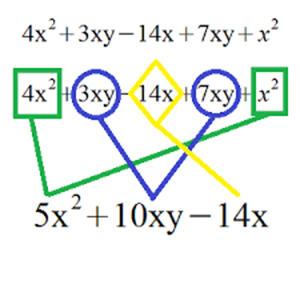In order to solve equations, students must understand the sorting and classifying of like terms. Students must be able to use the distributive property proficiently. This is a skill that students must master in order to proceed and be successful in higher mathematics. The fundamentals for combining like terms begins in elementary school with sorting and classifying shapes and objects. Combining like terms is a continuation of developing these skills.

#### 33 Combining Like Terms Practice Review Part 2 02:30

This video reviews the second part of the Practice for "Combining Like Terms", Lesson 12. The practice review consists of two videos. Make sure that you complete Part I first, then use this video to finish the review.

#### 34 Pre-Algebra Part II 16:34Using multiplication and division to solve equations is the focus on this lesson. Mastering solving equations is imperative to pre-algebra and beyond. The objective is to give students time to master each part of the algorithm before introducing more content.

#### 35 PreAlgebra II Practice Part One 02:20

This video reviews the lesson practice for PreAlgebra II in two parts. This video is for Part I of the practice. Use this video to evaluate your work and correct any errors that you may have made. Make certain that you understand your mistakes. Hoping that you were successful!

#### 36 PreAlgebra II Practice Part Two 06:00

This is part two of the review for the Practice for PreAlgebra II. Make sure that you have already reviewed Part I before going to this practice video.

#### 37 Pre-algebra II Part 3 06:00

Review your Practice 3 from "PreAlgebra II". Make certain to review any errors that you made and be certain to understand your errors. These are important skills to have mastered before we move on so make sure that you have mastered these skills. Take time to solve any examples that you may have missed. Good luck.

#### 38 Pre-Algebra III 10:48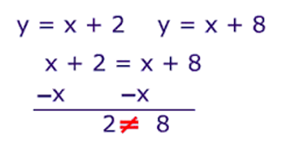Students will solve equations using all four operations. Students will continue to practice and master the skills for solving algebraic equations including fractions and decimals.

#### 39 Prealgebra III Practice 07:45

Students will assess their practice for combining like terms.

#### 45 Solving Multiple Step Equations 10:48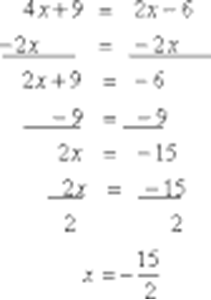Students will extend concepts of linear equations and inequalities in one variable to more complex multi-step equations and inequalities in real-world and mathematical situations.

#### 46 Solving Multiple Step Equations Practice 10:48

Students will use the video to assess their mastery of solving multiple step equations. Students need to practice and make certain that they understand solving these equations.

#### 40 Slippery Slopes 08:12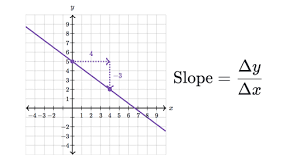Students are introduced to the concepts of slope using real-world examples. Students will apply the concepts of slope and y-intercept to graphs, equations, and proportional relationships.

#### 41 Slippery Slopes Practice 08:18

Students will use the video to help solve problems in the interactive quiz used for practice of writing equations in different forms to slope-intercept form. Good Luck. Remember to analyze any errors you made and find out what you did wrong.

#### 42 Slope Intercept Form 02:41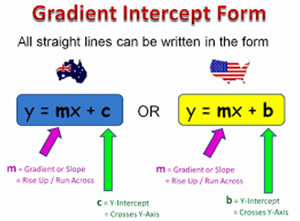Students will learn how to use slope-intercept form to identify a line, graph a line, and write the equation of a line. Students will apply concepts of slope and y-intercept to graphs, equations, and proportional relationships.

#### 43 Graphing Using Slope Intercept Form 04:19

Students will practice graphing linear equations using the slope-intercept form. The slope-intercept form requires students to graph the y intercept and use the slope to find the next points on the line.

#### 44 Slope Intercept Form Practice 07:04

This video reviews the exercise for graphing lines using slope intercept form. The video explains the exercise in detail and models how to graph the lines using this method.

#### 47 Graphing Three Methods 04:20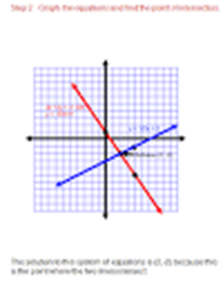Students will use one of three methods to graph linear equations. They can graph using slope-intercept form, point slope form, or using intercepts. This video demonstrates graphing linear equations in those 3 ways.

#### 48 Graphing Three Methods Practice 07:04

Use the video to evaluate your practice of graphing linear systems. Although the lesson talks about graphing with three methods, the practice only requires you to use slope intercept form.

Be sure to check your work and analyze your mistakes. Good luck.

#### 49 Linear Inequalites 14:13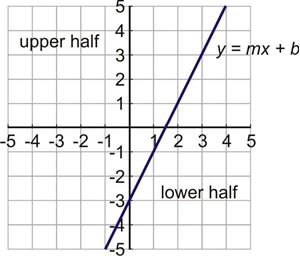Students will learn to evaluate, graph, and read linear inequalities on a number line. Solving linear inequalities is very similar to solving linear equations except the equal sign has been replaced by the greater than or less than symbols.

#### 50 Graphing Inequalities Practice 08:00

Students can use this video to evaluate their practice once it is completed. Make sure to look at your mistakes and be certain that you understand your misunderstaning. Good luck.

#### 51 Graphing with Intercepts Practice 04:19

Students will assess their skills of graphing with intercepts.

#### 52 Solving Systems of Equations 10:53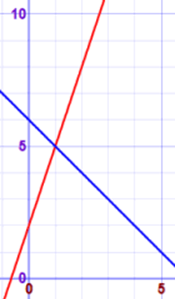Students will learn how to identify the point that is a solution for a variety of lines on a graph.

#### 53 Solving Systems of Equations Practice 04:24

Students will use the video to assess their understanding of graphing systems of equations.

#### 54 Pythagorean Theorem 17:47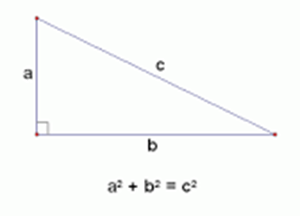Students will apply the Pythagorean Theorem to model and solve real world and mathematical problems in two and three dimensions involving right trainagles.

#### 55 Transformations 25:40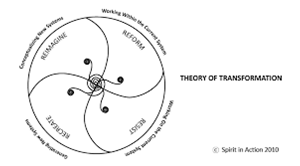Students will investigate the properties of rigid transformation (rotations, reflections, translations) using a variety of tools (e.g. grid paper, reflective devices, graphing paper, technology). Apply the properties of rigid transformations and investigate the properties of transforamtions using a variety of tools. Apply the properties of dilations.

#### 56 Transformations Practice 1 06:06

Students will use the video to assess their success with transfornations. Good Luck!

#### 57 Transformations II 25:51This lesson demonstrates to students what happens to the ordered pair when a line is reflected, rotated, translated, or dilated across the axis. Students need to be able to refelct, rotate, translate or dilate across a specific line.

#### 58 Transformations II Practice Review 05:39

Students will use the video to assess their mastery of transformations. Good Luck!

#### 59 Bivariate Data Lesson 09:07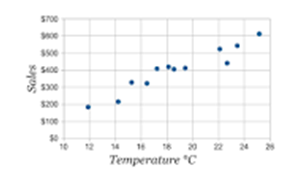Students will read, interpret, and make inferences based on the data from different tables. Students will investigate bivariate categorical data in two-way tables.

#### 60 Bivariate Data Lesson Practice 04:25

Students will use the data to evaluate their practice lesson that they have completed. Be sure to go back and look at any errors that you had and analyze what mistake you made. Good luck.

#### 61 Scatter Plots 08:40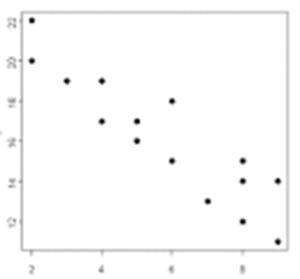Students will draw an approximate line of best fit on a scatter plot that appears to hae a linear association and informally assess the ift of the line to the data points.

#### 62 Scatter Plots Practice 08:35

Students will use the video to evaluate their mastery of scatterplots, creating the plots, making predictions from the plots, and reading the scatterplots. Good luck.

#### 63 Pythagorean Theorem Practice 05:39

Students will assess their success with finding the missing lengths of a right triangle by using the Pythagorean Theorem.

#### 64 Prealgebra Final Exam 00:00

The pre-algebra final exam will assess a student's mastery of pre-algebra skills. If you have difficulty with any part of the exam, you can always review those skills and try again. The answer key for the final exam can be found under resources.

Course Overview

This course is designed to develop an instructional approach that addresses the needs of middle grades students with a variety of ability levels and learning styles. This course is a program that brings math to life. It integrates mathematical strands making mathematical connections real. It offers numerous opportuniites for students to develop problem solving, critical thinking, data analysis, and communication skills. This course, along with the assignments given is aligned with eighth grade math. We will emphasize critical thinking, problem  solving, real-world applications, and the use of technology.

This course includes:

• 5 units - REAL NUMBERS, ALGEBRAIC EQUATIONS, LINEAR EQUATIONS AND GEOMETRY
• WORKSHEETS WITH VIDEO REVIEW OF EACH PRACTICE
• 30 VIDEO LESSONS
• 30 EXERCISE REVIEW LESSONS
• 54 LINKS TO INTERACTIVE WEBSITES TO PRACTICE CONCEPTS
• 46 PRACTICE LESSONS
• 2 Tests
• 4 Answer Keys for Test

Course Goals

Upon completion of this course students will be prepared to enter Algebra I.

This course is designed for students prepared for Pre-Algebra.

This video course is primarily intended for seventh and eighth grades who have mastered basic math skills.

Course Requirements

Students taking this course will need to have mastered basic math skills, numeracy, and developed critical thinking skills.

Course Topics

Unit I - Real Numbers

Students will learn how to effectively take notes and use notes to increase retention of new material.

• A lesson on how to take notes.
• How to complete a first and second repeat on notes.
• How to write a summary.
• How to use notes.

Real Numbers - Explore the real number system and its appropriate usage in real-world situations.

• Rational Numbers - Estimate and compare the value of irrational numbers by plotting them on a number line. Estimate and compare the value of irrational numbers by plotting them on a number line. Fractions, Decimals, and Percent - Extension of rational numbers extend prior knowledge to translate among multiple representations of rational numbers (fractions, decimal numbers, percentages). Apply the concepts of proportional relationships to real-world and mathematical situations. Understand and apply the laws of exponents (i.e., product rule, quotient rule, power to a power, product to a power, quotient to a power, zero power property, negative exponents) to simplify numerical expressions that include integer exponents. Understand and apply the laws of exponents (i.e., product rule, quotient rule, power to a power, product to a power, quotient to a power, zero power property, negative exponents) to simplify numerical expressions that include integer exponents. Apply concepts of proportional relationships to real-world and mathematical situations. Investigate concepts of square and cube roots. Apply concepts of decimal and scientific notation to solve problems. Explore the relationship between quantities in decimal and scientific notation.

Unit Two - Algebraic Equations

Use variables to represent quantities in a real-world or mathematical problem, and construct simple equations and inequalities to solve problems through reasoning. Use properties of numbers to solve equations.Solve equations fluently. Solve Equations fluently.

Unit Three

Solve Equations fluently. Solve multi-step equations. Understand solving with variables on both sides of the equation. Understand properties of numbers and use properties to solve problems. Use algebraic thinking to solve real-world problems. Write and solve algebraic equations for real-world problems.

Unit Four

Extend concepts of linear equations and inequalities in one variable to more complex multi-step equations and inequalities in rel-world and mathematical situations. Understand slope, intercepts, graphing on coordinate plane, solve graphically and algebraically, identify different types of linear equations by their properties, solve by elimination, substitution, and graphically. Apply the concepts of slope and y-intercept to graphs, equations, and proportional relationships. Explore the concept of functions. Extend concepts of linear equations and inequalities in one variable to more complex multi-step equations and inequalities in real-world and mathematical situations. Investigate and solve real-world and matematical problems involving systems of linear equations in two variables with integer coefficients and solutions. Investigate and solve real-world and mathematical problems involving systems of linear equations in two variables with integer coefficients and solutions.

Unit 5

Investigate bivariate categorical data in two-way tables. Draw an approximate line of best fit on a scatter plot that appears to have a linear association and informally assess the fit of the line ot the data points. Investigate the properties of rigid transformations (rotations, reflections, translaations) using a variety of tools (e.g., grid paper, reflective devices, graphing paper, technology). Use models to demonstrate a proof of the Pythagorean Theorem and its converse. Apply the properties of rigid transformations (rotations, reflections, translations). Investigate the properties of rigid transformations (rotations, reflections, translations) using a variety of tools (e.g., grid paper, refelctive devices, graphing paper, technology). Investigate the properties of rigid transformation using a variety of tools.

• Teacher: Sheila
• Areas of expertise: Mathematics
• Education: BS, MAED, ED.S.
• Interests: Nature, outdoor activities, survival,
• Skills: My greatest talent as a teacher is providing my students with differentiated instruction that meets their needs. I provide my students with a plethora of learning opportunities that increases their understanding of mathematical concepts and builds their skills. I enjoy watching my students grow and the more successful they become, the more successful that I feel. I continually strive to provide the best instruction possible to my students.
• Associations: I am a member of SCAMT,, South Carolina Association of Math Teachers, and the National Mathematical Association of Math Teachers.
• Issues I care about: I want my students to have the skills necessary to have a successful future in whatever endeavors they choose. I feel an obligation to give my students opportunities that will allow them to thrive and build a good life for themselves. Most of my students live in poverty with only one parent or with a grandparent. I want my students to be able to break down the barrier of poverty, to overcome obstacles and change their world.

I have devoted more than 30 years to teaching and still enjoy each day with my students. Teaching is about more than just providing students with knowledge about math, it is about preparing them to be successful in a world that is rapidly changing. Technology is increasing the possibilities for careers in fields that we do not even know about yet. I want my students to be prepared to be a part of that new world, to feel confident in their abilities, and to be life-long independent learners. Even though it sounds like a cliche', there is nothing more true than the fact that these children are our future.

Find the answer key here for Transformations.

#### Answer Key For Quiz On Real Numbers

Interactive Quiz on Real Numbers for students to use to evaluate their mastery.

#### ANSWER KEY FOR FINAL EXAM

You will find the answers for the final exam here.

#### Answer Key for Quiz on Monomials

Students can assess their mastery of monomials using this online quiz.

Customers who bought this item also boughtThis is a full-year Algebra 1 course and it is the critical element in giving students a solid foundation for all future mathematics courses through their post-secondary education. The course can be aligned to most states' Common Core curriculum and is perfect for homeschool groups. +
\$249.00 \$119.00This course provides a basic overview of the skills necessary to succeed in Algebra 1. Topics include solving equations, graphing linear equations, solving inequalities and graphing linear inequalities. +
\$199.00 \$95.00This course covers a full year of 8th grade mathematics curriculum including: Number Operations, Geometric concepts, Pre-algebra, Data Management, and Probability. +
\$179.00 \$85.00